# Redis数据淘汰算法

``````maxmemory 1G
``````

• `volatile-lru`: 最近最少使用算法，从设置了过期时间的键中选择空转时间最长的键值对清除掉

• `volatile-lfu`: 最近最不经常使用算法，从设置了过期时间的键中选择某段时间之内使用频次最小的键值对清除掉

• `volatile-ttl`: 从设置了过期时间的键中选择过期时间最早的键值对清除

• `volatile-random`: 从设置了过期时间的键中，随机选择键进行清除

• `allkeys-lru`: 最近最少使用算法，从所有的键中选择空转时间最长的键值对清除

• `allkeys-lfu`: 最近最不经常使用算法，从所有的键中选择某段时间之内使用频次最少的键值对清除

• `allkeys-random`: 所有的键中，随机选择键进行删除

• `noeviction`: 不做任何的清理工作，在redis的内存超过限制之后，所有的写入操作都会返回错误；但是读操作都能正常的进行

``````maxmemory_policy volatile-lru
``````

### LRU算法

LRU是 `Least Recently Used` 的缩写，即最近最少使用，很多缓存系统都使用此算法作为淘汰策略。### Redis的LRU算法

Redis使用了结构体 `robj` 来存储缓存对象，而 `robj` 结构有个名为 `lru` 的字段，用于记录缓存对象最后被访问的时间，Redis就是以 `lru` 字段的值作为淘汰依据。`robj` 结构如下：

``````  redisObject {
...
lru:;
...
} robj;
``````

``````robj *(redisDb \*db, robj \*key,  flags) {
dictEntry *de = (db->,key->);
(de) {
robj *val = (de);

(server. == - &&
server. == - &&
!(flags & LOOKUP_NOTOUCH))
{
(server. & MAXMEMORY_FLAG_LFU) {
(val);
}  {
val-> = ();
}
}
val;
}  {
;
}
}
``````

`lookupKey()` 函数用于查找key对应的缓存对象，所以当缓存对象被访问时便会调用此函数。

### Redis数据淘汰

Redis在处理每一个命令时都会检查内存的使用是否超过了限制的最大值，处理命令是通过 `processCommand()` 函数进行的，检查内存使用情况的代码如下：

`````` (client *c) {
...
(server. && !server.) {
out_of_memory = () == C_ERR;

(server. == )  C_ERR;

(out_of_memory &&
(c->-> & CMD_DENYOOM ||
(c-> & CLIENT_MULTI && c->-> != execCommand))) {
(c);
(c, shared.);
C_OK;
}
}
...
}
``````

`````` () {
...
mem_reported, mem_tofree, mem_freed;
latency, eviction_latency;
delta;
slaves = (server.);
...
((&mem_reported,,&mem_tofree,) == C_OK)
C_OK;

mem_freed = ;

(server. == MAXMEMORY_NO_EVICTION)
cant_free;
``````

`freeMemoryIfNeeded()` 函数首先会调用 `getMaxmemoryState()` 函数来获取Redis的内存使用情况，如果 `getMaxmemoryState()` 函数返回 `C_OK`，表示内存使用总量还没有超出限制，直接返回 `C_OK` 就可以了。如果 `getMaxmemoryState()` 函数不是返回 `C_OK`，表示内存使用总量已经超出限制，需要进行数据淘汰，需要淘汰数据的大小通过 `mem_tofree` 参数返回。

``````    (latency);
(mem_freed < mem_tofree) {
j, k, i, keys_freed = ;
next_db = ;
sds bestkey = ;
bestdbid;
redisDb *db;
dict *dict;
dictEntry *de;

(server. & (MAXMEMORY_FLAG_LRU|MAXMEMORY_FLAG_LFU) ||
server. == MAXMEMORY_VOLATILE_TTL)
{
evictionPoolEntry *pool = EvictionPoolLRU;

(bestkey == ) {
total_keys = , keys;

(i = ; i < server.; i++) {
db = server.+i;
dict = (server. & MAXMEMORY_FLAG_ALLKEYS) ?
db-> : db->;
((keys = (dict)) != ) {
(i, dict, db->, pool);
total_keys += keys;
}
}
(!total_keys) ;

(k = EVPOOL_SIZE-; k >= ; k--) {
(pool[k]. == ) ;
bestdbid = pool[k].;

(server. & MAXMEMORY_FLAG_ALLKEYS) {
de = (server.[pool[k].].,
pool[k].);
}  {
de = (server.[pool[k].].,
pool[k].);
}

(pool[k]. != pool[k].)
(pool[k].);
pool[k]. = ;
pool[k]. = ;

(de) {
bestkey = (de);
;
}  {

}
}
}
}
``````

``````          (server.maxmemory_policy == MAXMEMORY_ALLKEYS_RANDOM ||
server.maxmemory_policy == MAXMEMORY_VOLATILE_RANDOM)
{
(i = ; i < server.; i++) {
j = (++next_db) % server.;
db = server.+j;
dict = (server. == MAXMEMORY_ALLKEYS_RANDOM) ?
db-> : db->;
((dict) != ) {
de = (dict);
bestkey = (de);
bestdbid = j;
;
}
}
}
``````

``````         (bestkey) {
db = server.+bestdbid;
robj *keyobj = (bestkey,(bestkey));
(db,keyobj,server.);

delta = ( ) ();
(eviction_latency);

(server.)
(db,keyobj);

(db,keyobj);
(eviction_latency);
(,eviction_latency);
(latency,eviction_latency);
delta -= ( ) ();
mem_freed += delta;
server.++;
(NOTIFY_EVICTED, ,
keyobj, db->);
(keyobj);
keys_freed++;

(slaves) ();

(server. && !(keys_freed % )) {
((,,,) == C_OK) {
mem_freed = mem_tofree;
}
}
}
``````

### 淘汰数据样本采集

`````` ( dbid, dict \*sampledict, dict \*keydict,  evictionPoolEntry *pool) {
j, k, count;
dictEntry *samples[server.];

count = (sampledict,samples,server.);
``````

`evictionPoolPopulate()` 函数首先调用 `dictGetSomeKeys()` 函数从缓存对象集合中获取一些样本，并保存在 `samples` 数组中。

``````     (j = ; j < count; j++) {
idle;
sds key;
robj *o;
dictEntry *de;

de = samples[j];
key = (de);

(server. != MAXMEMORY_VOLATILE_TTL) {
(sampledict != keydict) de = (keydict, key);
o = (de);
}

(server. & MAXMEMORY_FLAG_LRU) {
idle = (o);
}   (server. & MAXMEMORY_FLAG_LFU) {
idle = -(o);
}   (server. == MAXMEMORY_VOLATILE_TTL) {
idle = ULLONG_MAX - ()(de);
}  {
();
}
``````

``````        k = ;
(k < EVPOOL_SIZE &&
pool[k].key &&
pool[k].idle < idle) k++;

(k ==  && pool[EVPOOL_SIZE-].key != ) {
;
}   (k < EVPOOL_SIZE && pool[k].key == ) {
}  {
(pool[EVPOOL_SIZE-]. == ) {
sds cached = pool[EVPOOL_SIZE-].;
(pool+k+,pool+k,
(pool[])*(EVPOOL_SIZE-k-));
pool[k]. = cached;
}  {
k--;
sds cached = pool[].;
(pool[]. != pool[].) (pool[].);
(pool,pool+,(pool[])*k);
pool[k]. = cached;
}
}

klen = sdslen(key);
(klen > EVPOOL_CACHED_SDS_SIZE) {
pool[k]. = (key);
}  {
(pool[k].,key,klen+);
(pool[k].,klen);
pool[k]. = pool[k].;
}
pool[k].idle = idle;
pool[k].dbid = dbid;
}
}
``````##### 热门推荐
•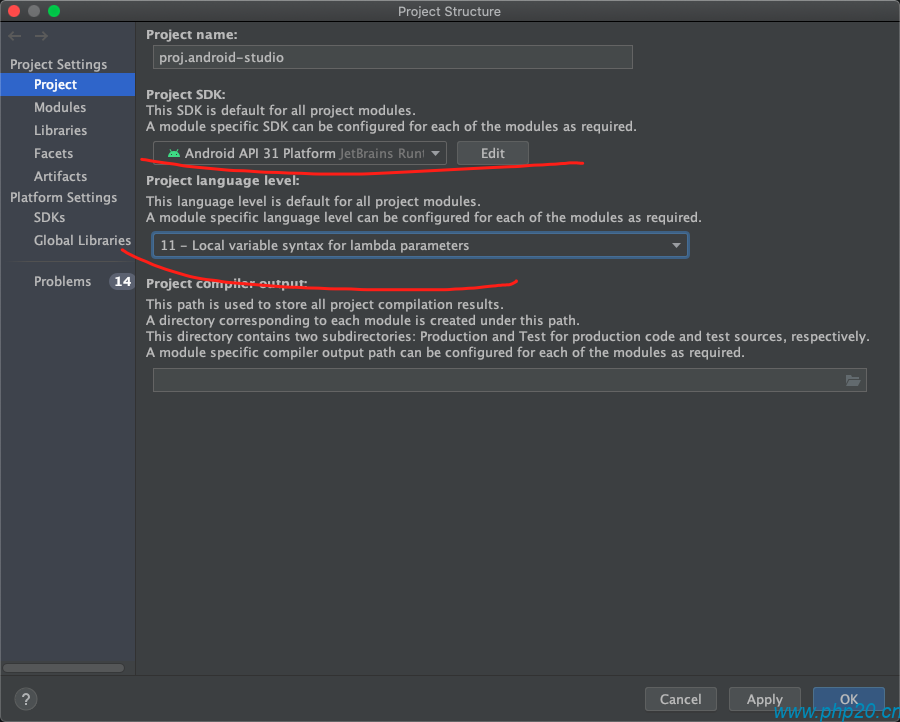浏览(1,986)
•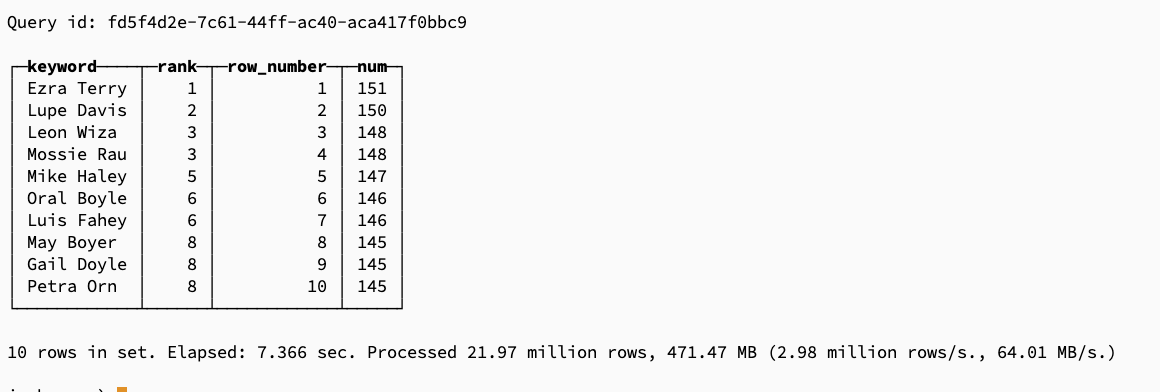浏览(791)
•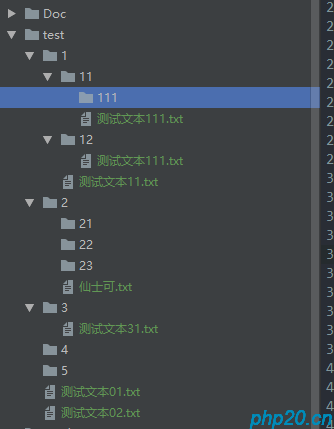浏览(782)
••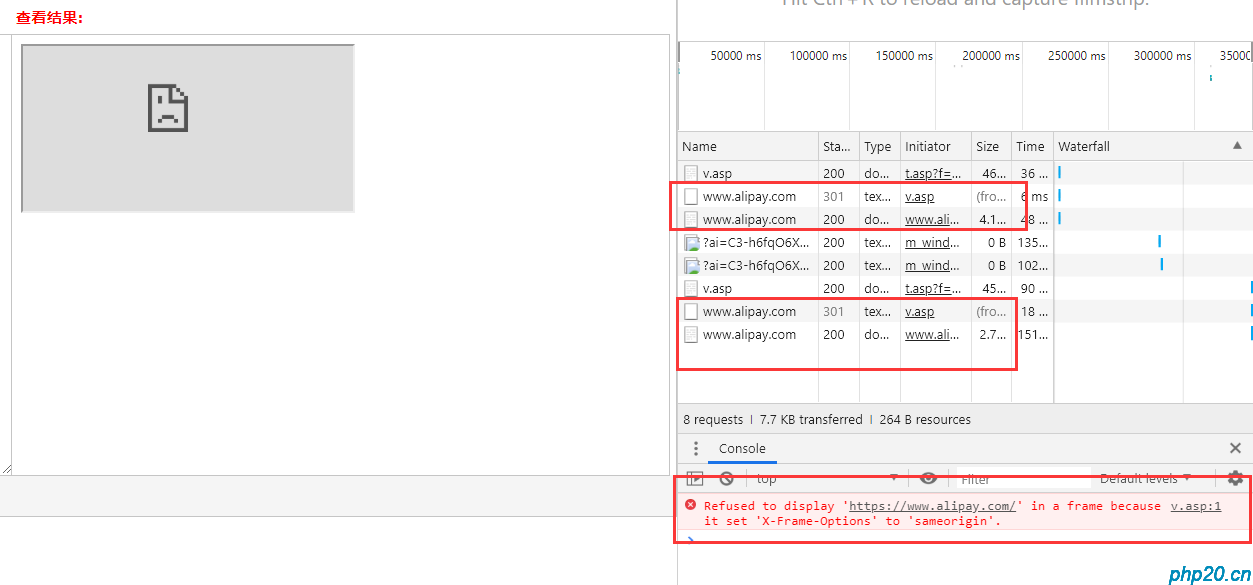浏览(685)
•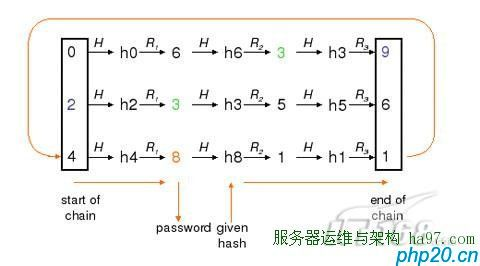•浏览(641)
•浏览(539)
•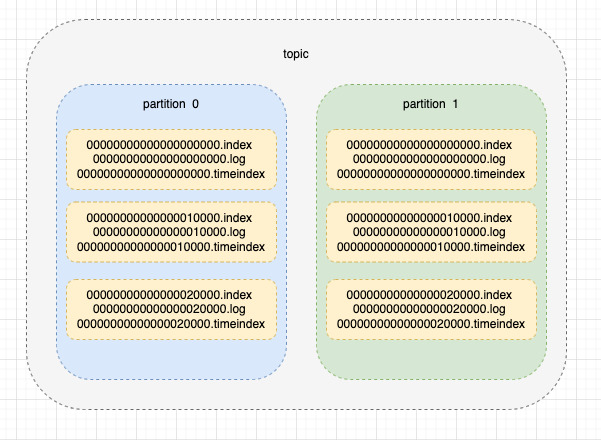浏览(487)
•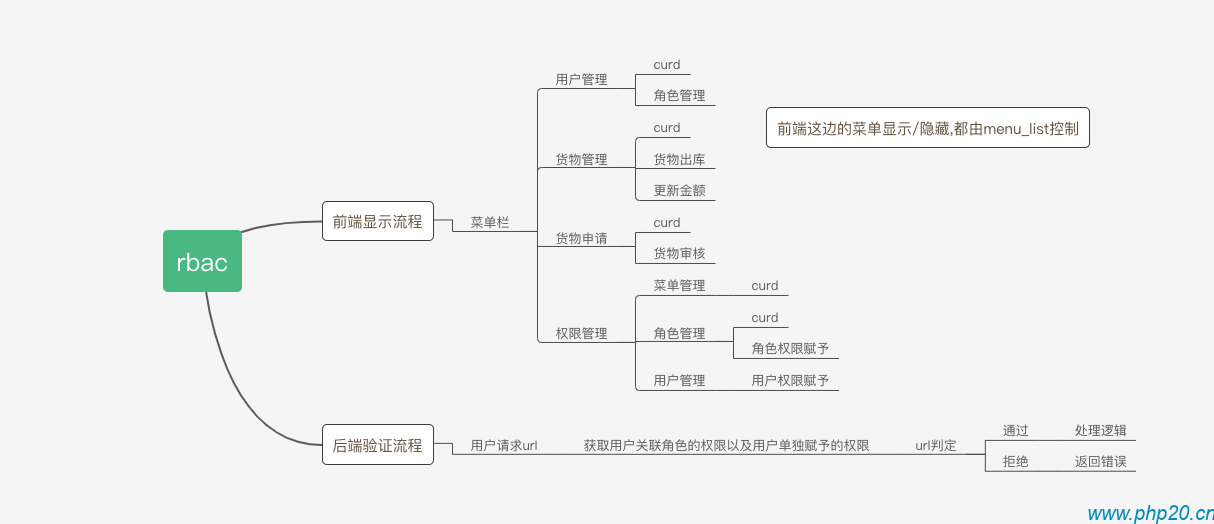浏览(477)